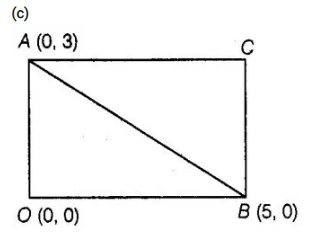# If AOBC is a rectangle whose three vertices

Question:

If AOBC is a rectangle whose three vertices are A(0, 3), O(0, 0) and B(5, 0), then the length of its diagonal is

(a) 5

(b) 3

(c) √34

(d) 4

Solution:Now, length of the diagonal AB = Distance between the points A(0, 3) and B(5, 0).

∴ Distance between the points (x,, y,) and (x2, y2),

$d=\sqrt{\left(x_{2}-x_{1}\right)^{2}+\left(y_{2}-y_{1}\right)^{2}}$

Here, $x_{1}=0, y_{1}=3$ and $x_{2}=5, y_{2}=0$

$\therefore$ Distance between the points $A(0,3)$ and $B(5,0)$

$A B=\sqrt{(5-0)^{2}+(0-3)^{2}}$

$=\sqrt{25+9}=\sqrt{34}$

Hence, the required length of its diagonal is √34.